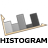You are here: Start » AVL.NET » Function Reference » Histogram » Histogram Combinators

# Histogram Combinators

Icon Name Description / Applications ModulesAddHistograms

Adds two histograms bar by bar.

FoundationBasicAddHistograms_OfArray

Adds histograms of an array bar by bar.

FoundationBasicAddHistograms_OfLoop

Adds histograms appearing in consecutive iterations bar by bar.

FoundationBasicDifferenceHistogram

Computes histogram representing difference between corresponding bars of given histograms.

FoundationBasicDivideHistograms

Divides two histograms bar by bar.

FoundationBasicMaximumHistogram

Computes the maximum of two histograms bar by bar.

FoundationBasicMaximumHistogram_OfArray

Computes the maximum of histograms of an array bar by bar.

FoundationBasicMaximumHistogram_OfLoop

Computes the maximum of histograms appearing in consecutive iterations bar by bar.

FoundationBasicMinimumHistogram

Computes the minimum of two histograms bar by bar.

FoundationBasicMinimumHistogram_OfArray

Computes the minimum of histograms of an array bar by bar.

FoundationBasicMinimumHistogram_OfLoop

Computes the minimum of histograms appearing in consecutive iterations bar by bar.

FoundationBasicMultiplyHistograms

Multiplies two histograms bar by bar.

FoundationBasicMultiplyHistograms_OfArray

Multiply histograms of an array bar by bar.

FoundationBasicMultiplyHistograms_OfLoop

Multiply histograms appearing in consecutive iterations bar by bar.

FoundationBasicSubtractHistograms

Subtracts two histograms bar by bar.

FoundationBasic